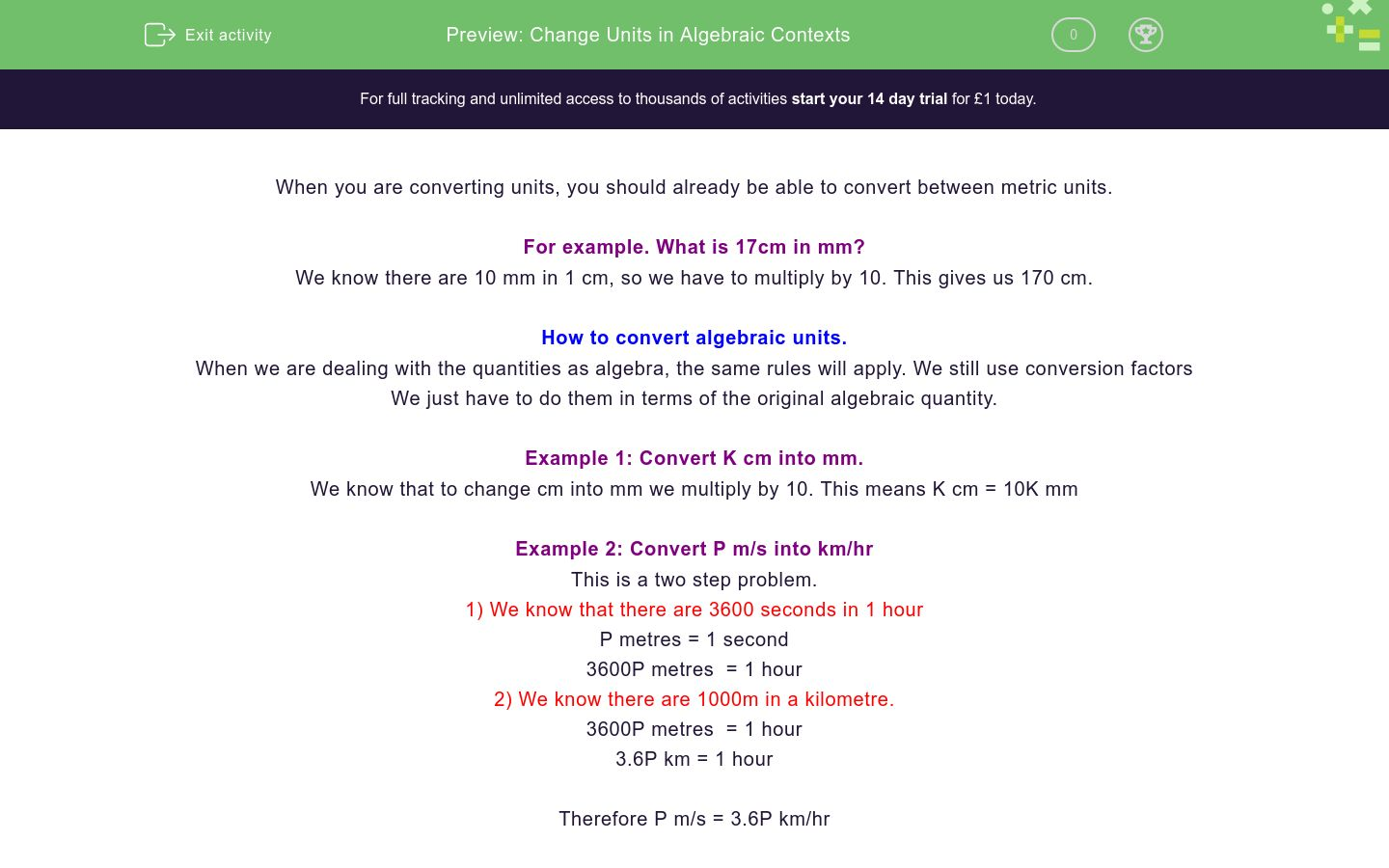# Change Units in Algebraic Contexts

In this worksheet, students practise changing algebraic units.Key stage:  KS 4

GCSE Subjects:   Maths

GCSE Boards:   AQA, Eduqas, Pearson Edexcel, OCR

Curriculum topic:   Ratio, Proportion and Rates of Change, Mensuration

Curriculum subtopic:   Ratio, Proportion and Rates of Change, Units and Measurement

Difficulty level:### QUESTION 1 of 10

When you are converting units, you should already be able to convert between metric units.

For example. What is 17cm in mm?

We know there are 10 mm in 1 cm, so we have to multiply by 10. This gives us 170 cm.

How to convert algebraic units.

When we are dealing with the quantities as algebra, the same rules will apply. We still use conversion factors

We just have to do them in terms of the original algebraic quantity.

Example 1: Convert K cm into mm.

We know that to change cm into mm we multiply by 10. This means K cm = 10K mm

Example 2: Convert P m/s into km/hr

This is a two step problem.

1) We know that there are 3600 seconds in 1 hour

P metres = 1 second

3600P metres  = 1 hour

2) We know there are 1000m in a kilometre.

3600P metres  = 1 hour

3.6P km = 1 hour

Therefore P m/s = 3.6P km/hr

When converting in algebraic contexts...

Convert P m into cm

100P

0.01P

Convert T cm into km.

Match the units

## Column B

T cm
0.000001T km
T cm
0.01T m
Tcm
10T mm

Convert R cm2 into mm2

Which of the following can be used to convert m/s into km/hr

x 3.6

÷ 3.6

x 18/5

x 3600/1000

x 5/18

Which of the following is the conversion for T g/cm3 in  kg/m3

1000T

0.1T

0.001T

Convert 36U km/hr into m/s

Convert D g/m<sup>3</sup> into kg/m<sup>3</sup>

Convert 750N kg/cm3 into g/cm3

• Question 1

When converting in algebraic contexts...

EDDIE SAYS
As we said in the intro When we are dealing with the quantities as algebra, the same rules will apply. We still use conversion factors We just have to do them in terms of the original algebraic quantity.
• Question 2

Convert P m into cm

100P
EDDIE SAYS
We know the conversion factor for m into cm is 100. As cm are smaller than m, there will be more of them for the same length so we have to multiply.
• Question 3

Convert T cm into km.

0.001T
0.001 T
EDDIE SAYS
The conversion factor here is 1000, if we divide T by 1000 we get...
• Question 4

Match the units

## Column B

T cm
0.01T m
T cm
10T mm
Tcm
0.000001T km
EDDIE SAYS
This is all about knowing your conversion factors centi = 100 milli - centi = 10 centi - kilo = 100 x 1000 = 100000
• Question 5

Convert R cm2 into mm2

100R
100 R
EDDIE SAYS
This is a bot more complicated. Changing cm into mm is x 10 but this is an area, so we need to multiply by 102
• Question 6

Which of the following can be used to convert m/s into km/hr

x 3.6
x 18/5
x 3600/1000
EDDIE SAYS
There's a number of ways we can state this. As we saw in the intro, multipying by 3600 and dividing by 1000 would give us the conversion factor. This can be written in three different ways that are all equivalent...
• Question 7

Which of the following is the conversion for T g/cm3 in  kg/m3

1000T
EDDIE SAYS
Firstly, we need to know the conversion factors for each. g - kg = ÷ 1000 Changing cm3 into m3 is not as easy as it looks. There is 100 cm in a metre but we are dealing with cubes. 1m3 = 100 x 100 x 100 = 1000000 cm3 If we tie these together, we get...
• Question 8

Convert 36U km/hr into m/s

10U
EDDIE SAYS
In the intro, we worked out that converting from m/s into km/hr was to multiply by 3.6. This means that doing it the other way round would be dividing by 3.6.
• Question 9

Convert D g/m<sup>3</sup> into kg/m<sup>3</sup>

1000D
EDDIE SAYS
As we previously worked out. Converting g/cm3 into kg/cm3 is a factor of 1000
• Question 10

Convert 750N kg/cm3 into g/cm3

0.75N
EDDIE SAYS
As we previously worked out. Converting g/cm3 into kg/cm3 is a factor of 1000 This means converting the other way round will be dividing by 1000
---- OR ----

Sign up for a £1 trial so you can track and measure your child's progress on this activity.

### What is EdPlace?

We're your National Curriculum aligned online education content provider helping each child succeed in English, maths and science from year 1 to GCSE. With an EdPlace account you’ll be able to track and measure progress, helping each child achieve their best. We build confidence and attainment by personalising each child’s learning at a level that suits them.

Get started